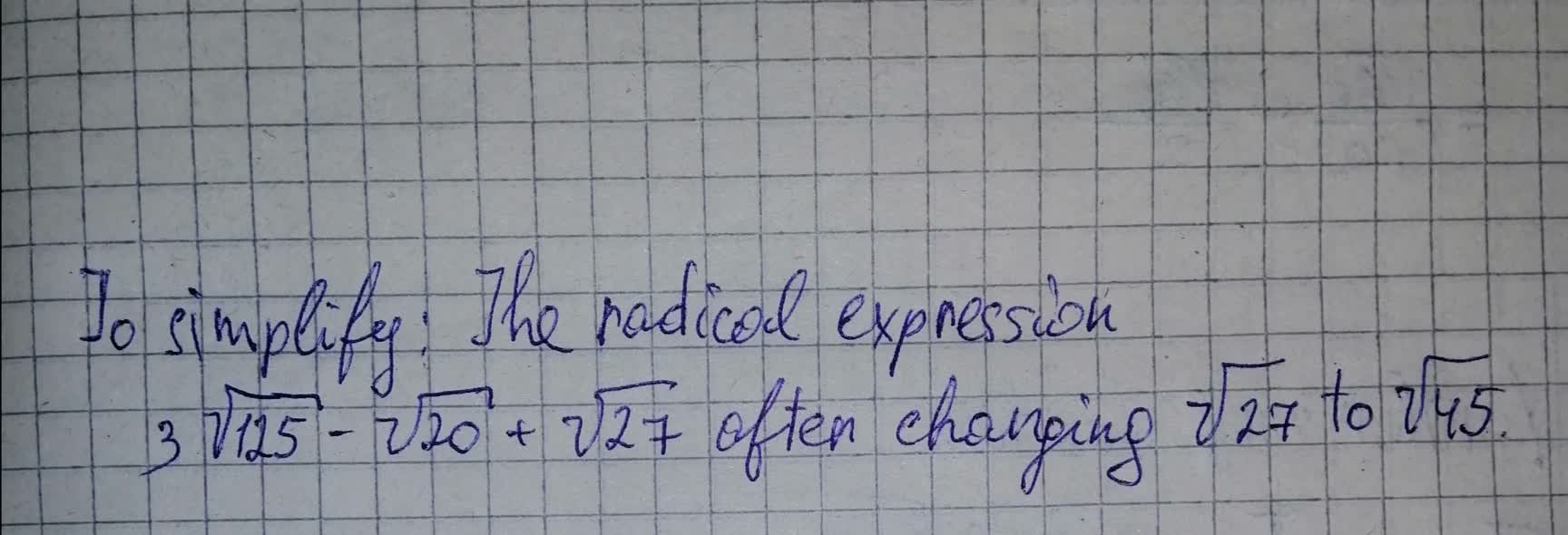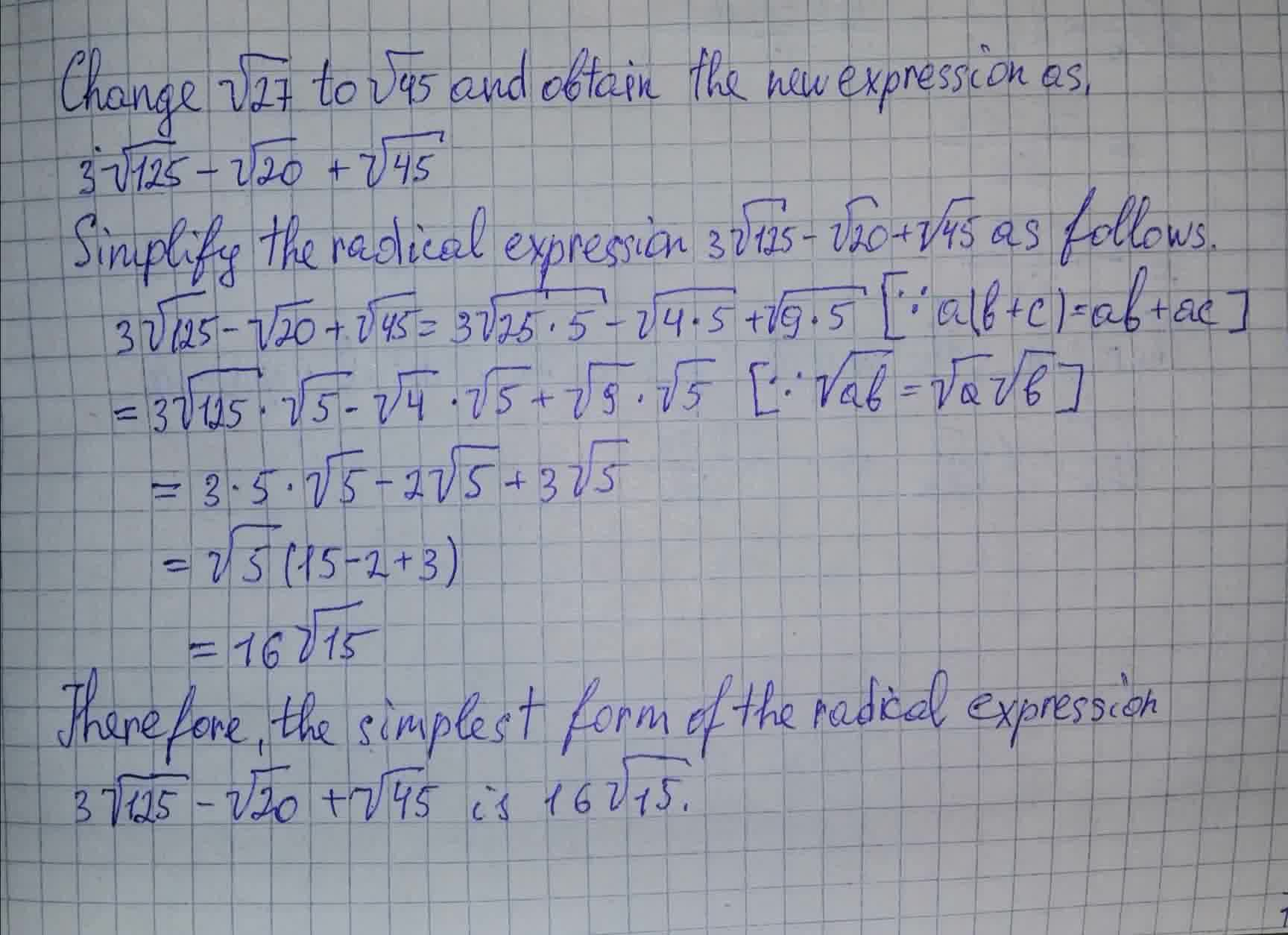Braxton Pugh 2021-07-30 Answered
Simplify radical expression $3\sqrt{125}-\sqrt{20}+\sqrt{27}$You can still ask an expert for help

• Questions are typically answered in as fast as 30 minutes

Solve your problem for the price of one coffee

• Math expert for every subject
• Pay only if we can solve itwornoutwomanC

Explanation:###### Not exactly what you’re looking for?

Simplify the following:
$3\sqrt{125}-\sqrt{20}+\sqrt{27}$

$\sqrt{125}=\sqrt{{5}^{3}}=5\sqrt{5}:$
$3×5\sqrt{5}-\sqrt{20}+\sqrt{27}$

$\sqrt{20}=\sqrt{{2}^{2}×5}=2\sqrt{5}:$
$3×5\sqrt{5}-2\sqrt{5}+\sqrt{27}$

Multiply 3 and 5 together.
$3×5=15:$
$15\sqrt{5}-2\sqrt{5}+\sqrt{27}$

$\sqrt{27}=\sqrt{{3}^{3}}=3\sqrt{3}:$
$15\sqrt{5}-2\sqrt{5}+3\sqrt{3}$

Add the numbers in $15\sqrt{5}-2\sqrt{5}+3\sqrt{3}$ together.
Add like terms. $15\sqrt{5}-2\sqrt{5}+3\sqrt{3}=3\sqrt{3}+13\sqrt{5}:$
Answer: $3\sqrt{3}+13\sqrt{5}$